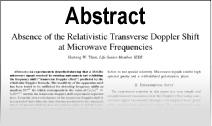Enter the content which will be displayed in sticky bar

AbstractPocklington Equation Method Versus Curved Segments Technique for the Numerical Study of Circular Antennas

Year: 2006 Pages: 14
Keywords: Curved segments, vector potential, Pocklington equation, method of moments.

We present a mathematical model applying the general Pocklington equation to arbitrary shaped thin wire antennas. In order to simplify the antenna analysis this approach uses the point matching technique and a simplified kernel form. By means of this technique it is possible to increase the Method of Moments solution convergence and reduce computational time and effort. To exemplity this the procedure is applied to the well-known circular loop antenna. The obtained results are compared with those of Champagne method, which uses quadratic segments, in order to get a numerical solution for the Electric Field Integral Equation.